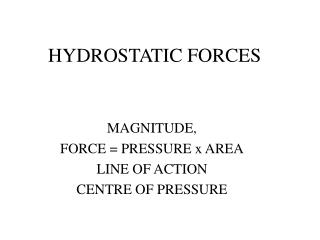DownloadDownload PresentationHYDROSTATIC FORCES

# HYDROSTATIC FORCES

Download Presentation## HYDROSTATIC FORCES

- - - - - - - - - - - - - - - - - - - - - - - - - - - E N D - - - - - - - - - - - - - - - - - - - - - - - - - - -
##### Presentation Transcript

1. HYDROSTATIC FORCES MAGNITUDE, FORCE = PRESSURE x AREA LINE OF ACTION CENTRE OF PRESSURE

2. HYDROSTATIC FORCE ON A HORIZONTAL SURFACE • CONSTANT PRESSURE • PASCAL’S PARADOX T W DAVIES

3. HYDROSTATIC FORCE ON VERTICAL SURFACES • INCLINED/VERTICAL SURFACES • PRESSURE CHANGES WITH DEPTH • NEED TO INTEGRATE OVER SURFACE T W DAVIES

4. MAGNITUDE OF HYDROSTATIC FORCE ON A PLAIN SURFACE • Force on a representative element • Integrate to give total force • Show that total force = wetted area x average pressure T W DAVIES

5. CENTRE OF PRESSURE • Turning moment on a representative element • Sum all such elements • Equate to moment of resultant force acting through centre of pressure T W DAVIES

6. CENTER OF PRESSURE • FULLY SUBMERGED SURFACE • top is y1 from free surface • bottom is y2 from free surface • I.e. h = y2 - y1 • ycp = 2/3 . (y23 - y13) / (y22 - y12) T W DAVIES

7. CENTRE OF PRESSURE • LIMITS • when y1 = 0, then y2 = h and ycp = 2/3. h • as submergence increases, ycp approaches ycg T W DAVIES

8. FORCES ON CURVED SURFACES • HORIZONTAL FORCE ON VERTICAL PROJECTION OF THE SURFACE, AVP • resultant force = pav . avp • c.o.p. as for plain surface • VERTICAL FORCE ON HORIZONTAL PROJECTION OF THE SURFACE, AHP • equal to weight of fluid contained or displaced • acts through centre of gravity of fluid mass T W DAVIES